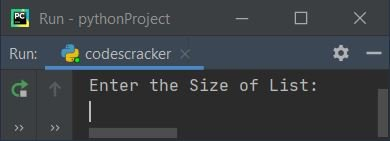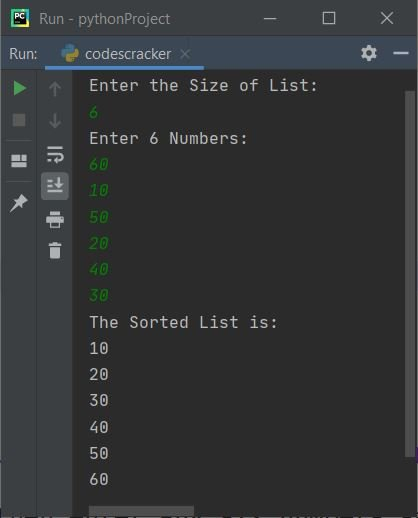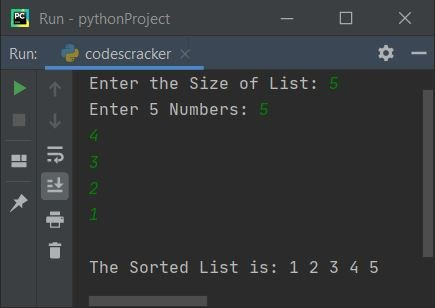# Bubble Sort Program in Python

In this article, you will learn and get code for bubble sorting in Python. But before going through the program on bubble sort, if you're not aware of the logic used behind it, refer to Bubble Sort Logic and Example to understand the topic.

## Bubble Sort using List

This program sorts a list using the bubble sort technique. The question is: write a program in Python to apply the bubble sort technique to sort a list. The answer to this question is given below:

```nums = []
print("Enter the Size of List: ")
tot = int(input())

print("Enter " + str(tot) + " Numbers: ")
for i in range(tot):
nums.insert(i, int(input()))

for i in range(tot-1):
for j in range(tot-i-1):
if nums[j]>nums[j+1]:
temp = nums[j]
nums[j] = nums[j+1]
nums[j+1] = temp

print("The Sorted List is:")
for i in range(tot):
print(nums[i])```

Here is its sample run:Now supply the size of the list, say 6, and then enter any six numbers, say 60, 10, 50, 20, 40, 30, and press the ENTER key to sort the list using the bubble sort technique as shown in the sample run given below:Note: The str() method is used to convert an int type value to a string type.

Note: The range() method returns a sequence of values. The value starts with 0 and increments by 1, by default. It stops before the number specified as its argument. For example, the following block of code

```for i in range(3):
print(i)```

prints 0 1 2 on output. The total execution of the print(i) statement is exactly three times the value given as an argument to range(). Each number is printed on a new line. Therefore, from the above program, the following statement:

`for i in range(tot-1):`

is used to execute the following block of code:

```for j in range(tot-i-1):
if nums[j]>nums[j+1]:
temp = nums[j]
nums[j] = nums[j+1]
nums[j+1] = temp```

(tot-i-1) number of times. In a similar way, the second for loop (the inner one) is used.

Since in bubble sort, each adjacent element gets compared and swapped if required, therefore, with this block of code, we've applied the bubble sort technique to sort the given list of numbers.

#### Modified version of the previous program

This is a modified version of the previous program. The only thing added to this program is the end to output the thing in Python. Since print() outputs the thing present inside its braces, it terminates with an automatic newline. Therefore, we've used end to end the thing with exactly what's given in its braces.

```nums = []
print(end="Enter the Size of List: ")
tot = int(input())

print(end="Enter " + str(tot) + " Numbers: ")
for i in range(tot):
nums.insert(i, int(input()))

for i in range(tot-1):
for j in range(tot-i-1):
if nums[j]>nums[j+1]:
temp = nums[j]
nums[j] = nums[j+1]
nums[j+1] = temp

print(end="\nThe Sorted List is: ")
for i in range(tot):
print(end=str(nums[i]) + " ")
print()```

Here is its sample run with user input 5 as size and 5, 4, 3, 2, and 1 as five numbers:Python Online Test

« Previous Program Next Program »